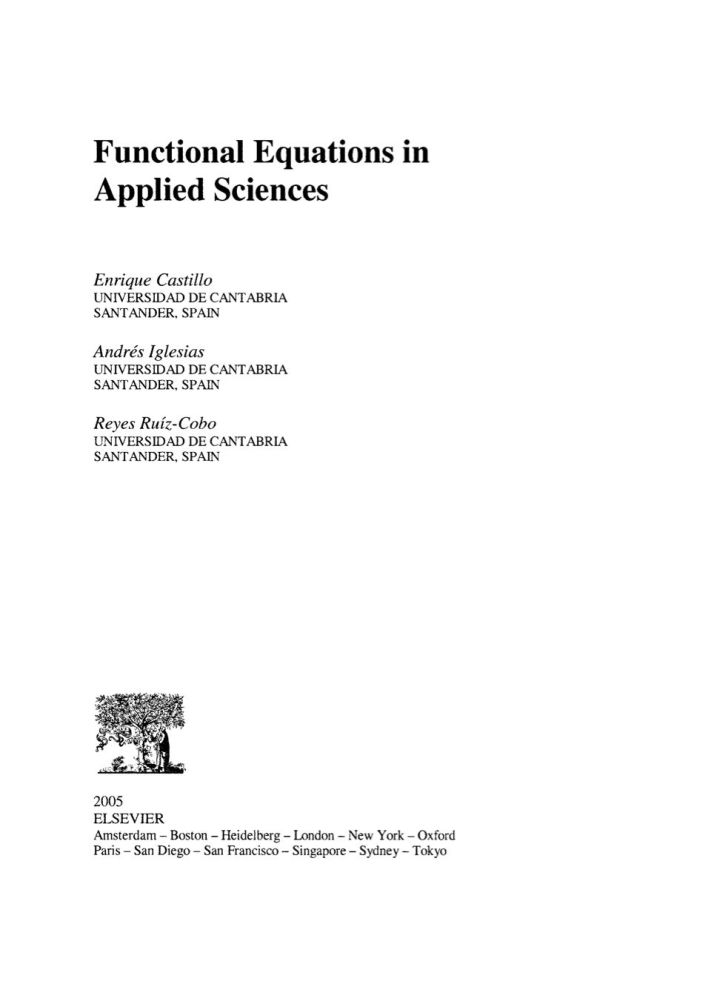# Functional Equations in Applied Sciences

## Functional Equations in Applied Sciences

By Castillo, Enrique; Iglesias, Andres; Ruiz-Cobo, Reyes

### Rent or Buy eTextbook

Expires on Apr 16th, 2022
\$255

Publisher List Price: \$279.99
Savings: \$24.99

The book provides the reader with the different types of functional equations that s/he can find in practice, showing, step by step, how they can be solved.
A general methodology for solving functional equations is provided in Chapter 2. The different types of functional equations are described and solved in Chapters 3 to 8. Many examples, coming from different fields, as geometry, science, engineering, economics, probability, statistics, etc, help the reader to change his/her mind in order to state problems as functional equations as an alternative to differential equations, and to state new problems in terms of functional equations or systems.
An interesting feature of the book is that it deals with functional networks, a powerful generalization of neural networks that allows solving many practical problems. The second part of the book, Chapters 9 to 13, is devoted to the applications of this important paradigm.
The book contains many examples and end of chapter exercises, that facilitates the understanding of the concepts and applications.

· A general methodology for solving functional equations is provided in Chapter 2.
· It deals with functional networks, a powerful generalization of neural networks.
· Many examples, coming from different fields, as geometry, science, engineering, economics, probability, statistics, etc, illustrate the concept of functional equation.
· Functional equations are presented as a powerful alternative to differential equations.
· The book contains end of chapter exercises.

Subject: Mathematics & Statistics -> Mathematics -> Mathematics GeneralPublisher: Elsevier S & T 11/2004
Imprint: Elsevier Science
Language: English
Length: 408 pages

ISBN 10: 044451788X
ISBN 13: 9780444517883
Print ISBN: 9780444517883

Live Chats